• Citation:  ES Energy & Environment, 2020, 7, 29-39

DOI:10.30919/esee8c361

Revised: 20 Nov 2020
Accepted: 26 Nov 2019
Published online: 26 Nov 2019

# Non-singular homogeneous polyhedral heat cloak and its realization

Qingxiang Ji,1,2 Xueyan Chen,1,2 Jun Liang,3 Vincent Laude,2 Sébastien Guenneau,4 Guodong Fang1 and Muamer Kadic2

1 National Key Laboratory of Science and Technology on Advanced Composites in Special Environments, Harbin Institute of Technology, Harbin 150001, China

2 Institut FEMTO-ST, CNRS, Université de Bourgogne Franche-Comté, Besançon 25000, France

3 Institute of Advanced Structure Technology, Beijing Institute of Technology, Beijing 100081, China

4 Aix−Marseille Univ, CNRS, Centrale Marseille, Institut Fresnel, Marseille, France

## Abstract

As most arbitrarily shaped cloaks can be approximated by polyhedra and further divided into a series of tetrahedra, we propose in this paper a linear mapping approach to design cloaks with tetrahedron shapes (i.e. tetrahedral cloaks). Homogeneous material properties of the cloak are straightforwardly obtained from coordinates of typical points. Consequently, most arbitrarily shaped thermal cloaks can be designed using homogeneous anisotropic materials only. We construct two polyhedral cloaks and show numerically that they successfully thermally conceal objects after a certain lapse of time. We then demonstrate that cloaks with curved boundaries can also be obtained using our approach. It is further shown how geometrical parameters affect the material properties and cloaking performances. One can ﬂexibly tune the cloaking performances based on practical requirements and material availabilities. We analyze effectiveness of a polygonal cloak composed of an alternation of homogeneous isotropic layers, and note that cloaking deteriorates at short times. We ﬁnally sketch an approach to realize 3D homogenized thermal metamaterials.

## Table of Content• Arbitrarily shaped homogeneous polyhedral cloaks were realized and the effectiveness was analysed.
• Homogenization of 3D thermal-metamaterials was sketched.

Keywords: heat cloaking; linear mapping transformation; arbitrarily shaped

## 1. Introduction

Heat cloaking refers to making an object invisible to heat ﬂows and is achieved by surrounding the object with a predeﬁned heat cloak. It is inspired by the initial works on invisibility of electromagnetic waves.1–3 Beyond electromagnetism,4-7 cloaking is widely applied to elastic waves,8-13 acoustics,14–16 and diffusion fields.17– 20 In our paper we focus on heat cloaking. Fan et.al.21 exploited shaped graded materials where heat energy was manipulated to transfer from regions of low temperature to regions of high temperature. Guenneau et.al.22 first proposed a transformed coordinate approach of thermal cloaking in the heat equation and established multi-layered structures with isotropic materials, which was veriﬁed later by experiments.23–26 Researchers have then made great achievements on heat manipulation with various thermal meta-devices.27–36 The pioneering works mainly focused on regular shaped cloaks, i.e. cylindrical and spherical cloaks. Cloaks with more complicated boundaries were then studied in the ﬁeld of electromagnetism and acoustics.37–44 These transforms were later introduced for heat diffusion ﬁelds.45 The obtained heat cloaks were inhomogeneous and anisotropic which is diffcult to realize in practice. Some researchers studied non regular-shaped cloaks and established systems with homogeneous parts,46-48 but they only dealt with limited geometries. Han et.al designed arbitrarily polygonal cloaks with homogeneous and non-singular parameters,49 which was realized by Liu et.al. with four kinds of natural bulk materials in bilayer conﬁguration throughout.50 Xu et.al. designed arbitrary polygonal thermal harvesting schemes using the linear mapping function.51-52 Li et.al. extended these works to three dimensions and proposed a multistep transformation approach for a tetrahedral cloak.53 However, in Li’s nice work, the transformation for a tetrahedral cloak is realized in three steps. The transformation should be conducted in a certain order as the later transformation step is dependent on the former one. We propose instead a one-step linear mapping for polyhedral cloaks where each transformation is independent. Homogeneous material parameters are obtained in this process, facilitating practical realizations. It is known that an arbitrary shaped cloak can be approached by polyhedra, but a quantitative analysis based on this approximation was rarely considered.50-53 We ﬁrst show how cloaks with curved boundaries (regular spherical cloak and cylindrical cloak as illustrative cases here) are approximated by polyhedra and we demonstrate the good cloaking performances of the approximated cloaks. For non-regular shaped cloaks, the convergence behavior in homogenization process hasn’t been studied, and this paper primarily focuses on this problem. We study the convergence behavior of a multi-layered polygonal cloak in both steady-state and transient state cases. Inﬂuences of the cloak’s shape and lapse of time on the convergence rate are numerically established. Finally, we propose an approach to realize 3D homogenized thermal metamaterials.

2. METHODS

We first consider a linear transformation from the tetrahedral domain in virtual space with local coordinate axes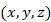to the physical tetrahedral space with local coordinate axes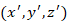(see Fig. 1) by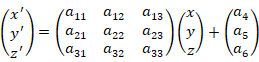,                   (1)

where the coefficients aij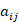and ah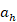are constant. Substituting four groups of corresponding vertices in Eq. 1, we get 12 equations for the 12 coefficients. By solving the 12 equations, we find the transformation coefficients as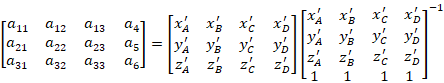.     (2)

The transient heat conduction equation without sources can be written as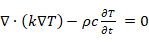,                     (3)

where T represents the temperature, k and ρc are thermal conductivity (in unit of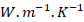) and the product of density by heat capacity (in unit of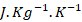), respectively. According to Ref. , this equation is form-invariant under coordinate transformation. Note that the transformed parameters are21-22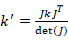and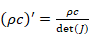,                       (4)

where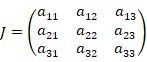.                           (5)

Now, the coefficient matrix Jis a constant matrix which is independent of x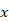, y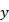and z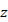. As a result, material properties derived from Eq. 4 are homogeneous.Fig. 1 Coordinate transformation between two tetrahedra. The tetrahedral domain in virtual space (left) is mapped to a physical tetrahedral space (right) by linear transformation.

In Fig. 2 we show a regular tetrahedral cloak presented by Ref. , where the shaded domain denotes the designed domain (cloaking domain) while tetrahedron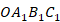represents the domain to be cloaked (cloaked domain). For clarity, we select the edge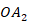to be along the x-axis, and the face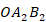in the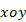plane. We further divide the polyhedral cloaking domain into three tetrahedral segments (marked in different colour in Fig. 2) and apply linear coordinate transformation to each segment. Tetrahedron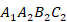,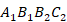and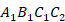in physical space are mapped from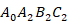,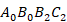and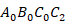in virtual space (outlined by green lines in Fig. 2), respectively. Since all the points are located on three edges, we define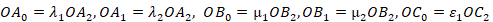and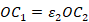, where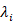,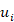and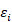are all known constants. In the transformation process, the physical space and virtual space are defined by the same coordinate system.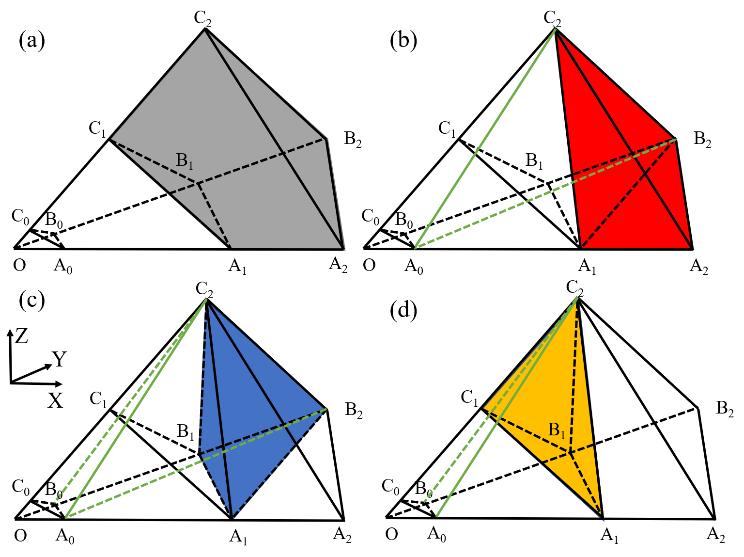Fig. 2 A general tetrahedron cloak and the sub-domains of the cloaking domain.

In Ref. , the transformation for a tetrahedron cloak is completed by multi-steps. The three segments should be mapped in a specific order as the latter transformation step is dependent on the former one. In our method, the transformations are all conducted by one step and each transformation is independent. Without losing generality, we first map the points in tetrahedronto the points in tetrahedron. Vertices of the given polyhedron in virtual space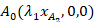,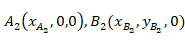and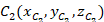are mapped to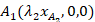,andin physical space, respectively. Substituting these four typical coordinates in Eq. 1, we get the transformation from tetrahedronto tetrahedronby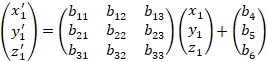,                     (6)

where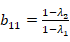,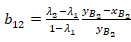,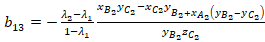,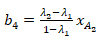,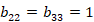,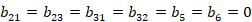.

Similarly, the points in tetrahedronare mapped onto tetrahedronby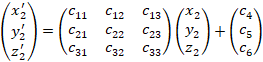,                (7)

where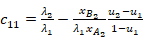,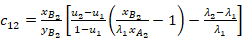,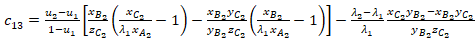,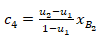,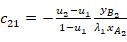,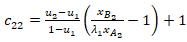,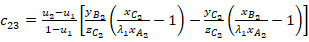,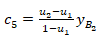,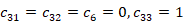.

The mapping from points in tetrahedronto points in tetrahedronis given by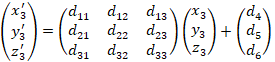,              (8)

where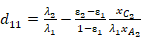,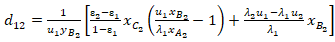,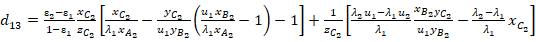,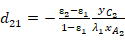,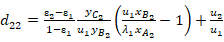,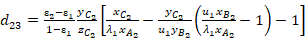,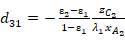,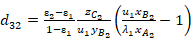,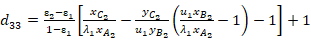,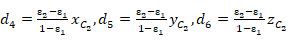Substituting the obtained transformation matrices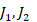and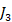into Eq. (4), we get the transformed material properties for each segment. In the above transformation, we assume the tetrahedron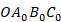is a void and we map it to the void tetrahedron, thus the object insideis cloaked. Remark that we can choose to fill the cloaked domain with a medium. Following Ref. , the transformed parameters for the cloaked domain are given by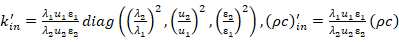.       (9)

## 3. Results and discussion

3.1  An octahedral cloak

We first build an octahedral cloak where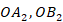and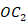lie on the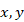andaxes, respectively (see Fig. (3a)). The domain to be cloaked is depicted in blue while the cloaking domain is depicted in the grey. We split the top and bottom halves into four symmetric tetrahedra. Each tetrahedron is divided into three segments and then mapped following the method outlined in above section. In the octahedral cloak, we set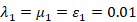and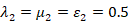. For convenience, we normalize the material properties with respect to unit conductivity and heat capacity in background medium. The octahedral cloak is totally divided into 8 tetrahedra and hence 24 segments. Owing to symmetry, we only present material properties of the three unique segments, which are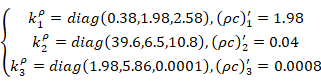Properties of other parts can be easily obtained using symmetries. Numerical simulations are then conducted with the finite element packag COMSOL Multiphysics. We set temperatures on the left boundary (the boundary in negative x axis) and right boundary (the boundary in positiveaxis) as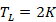and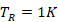, respectively. Other boundaries are thermally insulated to ensure that conduction is the dominant heat transfer mechanism in this case. For clarity, we only show the cloaking performances on two typical sections where the white lines are iso-thermal lines. Simulation results for the steady-state case (see Fig. (3b)) show that heat fluxes pass through the object and return to their original trajectory. As a result, the external heat field is not disturbed. For an outside observer, it seems that the heat is transferred through a homogeneous medium.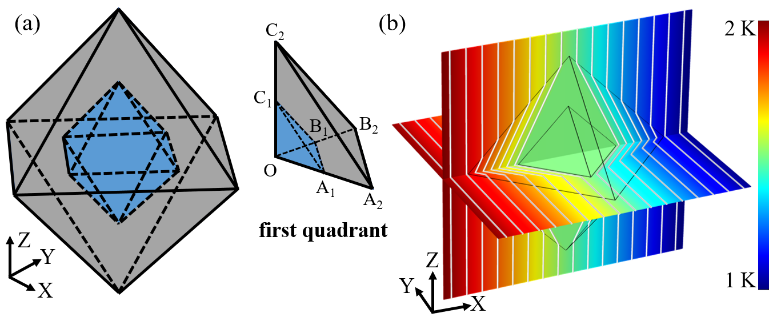Fig. 3 Schematic of (a) an octahedral heat cloak and (b) its steady-state cloaking performance.

In the dynamic case, we add perfectly matched layer on the right boundary and apply different time-dependent thermal sources on the left boundary, which are exp-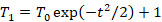and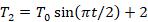. We set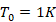and conduct simulations. Good cloaking performances are observed for both cases at different time. In Fig. 4, we set continuously decreasing temperatures on the left boundary and observe good cloaking effects all the times. In Fig. 5, we apply periodic thermal boundary on the left and notice that heat energy transfers from left to right. During the transfer process, good cloaking performance is observed at changing thermal profiles.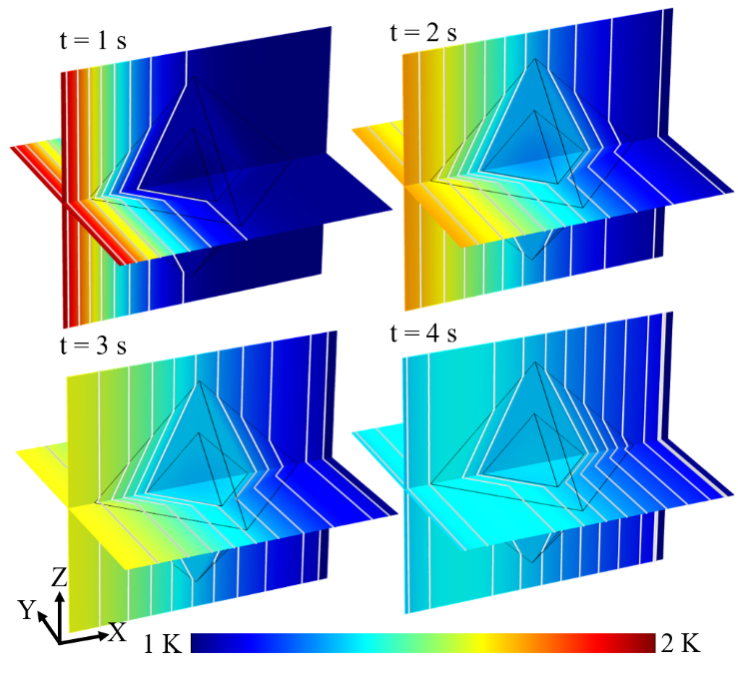Fig. 4 Dynamic cloaking performances of the octahedral cloak with the left boundary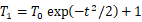.